# RS Aggarwal Solutions for Class 6 Maths Chapter 5 Fractions Exercise 5G

RS Aggarwal Solutions for Class 6 Maths Chapter 5 Fractions Exercise 5G has objective type questions which help students self analyse their overall knowledge about the Chapter. Important formulas and shortcuts which are used in solving problems are highlighted to make them easy for the students while studying. The solutions contains good explanation in order to help the students understand them quickly without any difficulty. Practising ample number of problems makes students perfect in solving them easily.

## Download PDF of RS Aggarwal Solutions for Class 6 Maths Chapter 5 Fractions Exercise 5G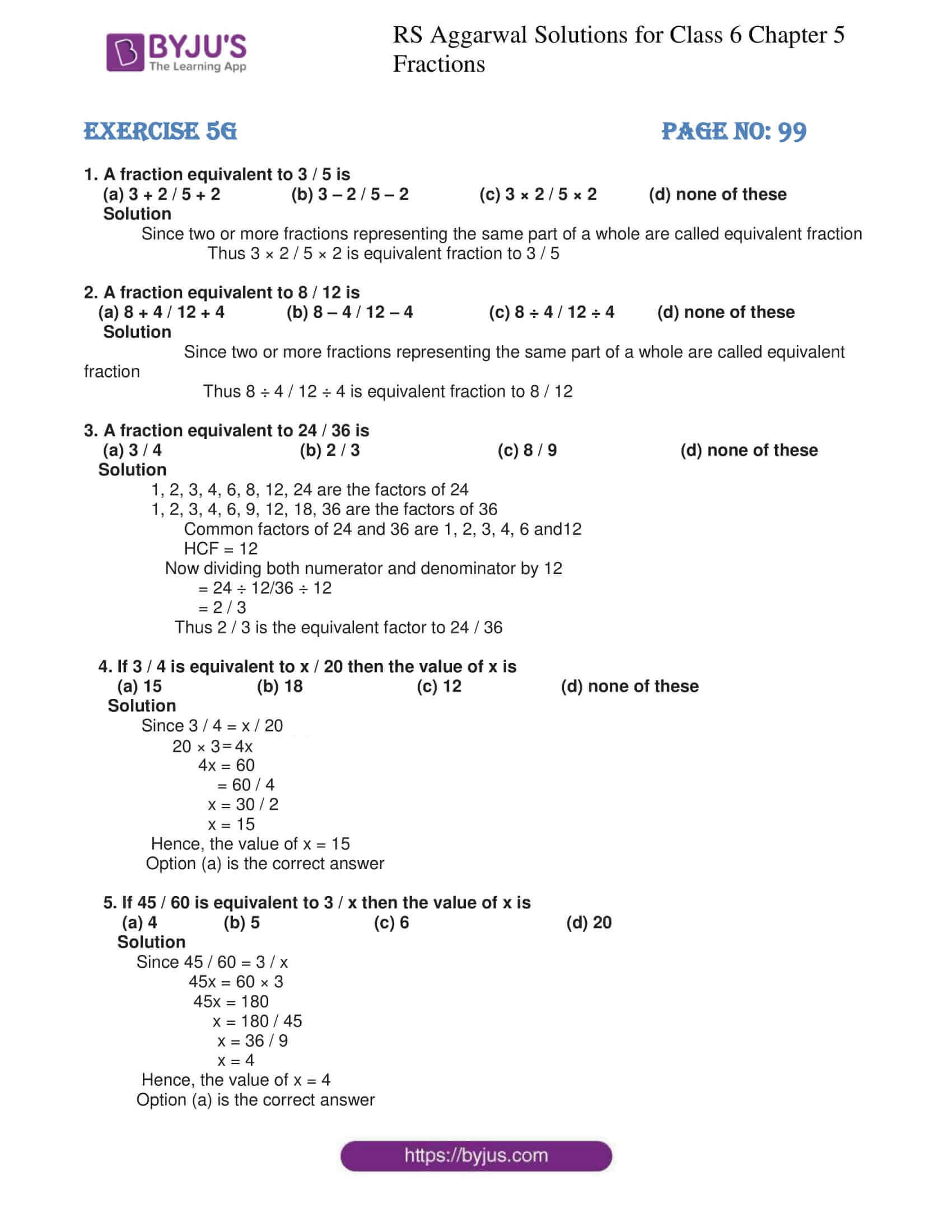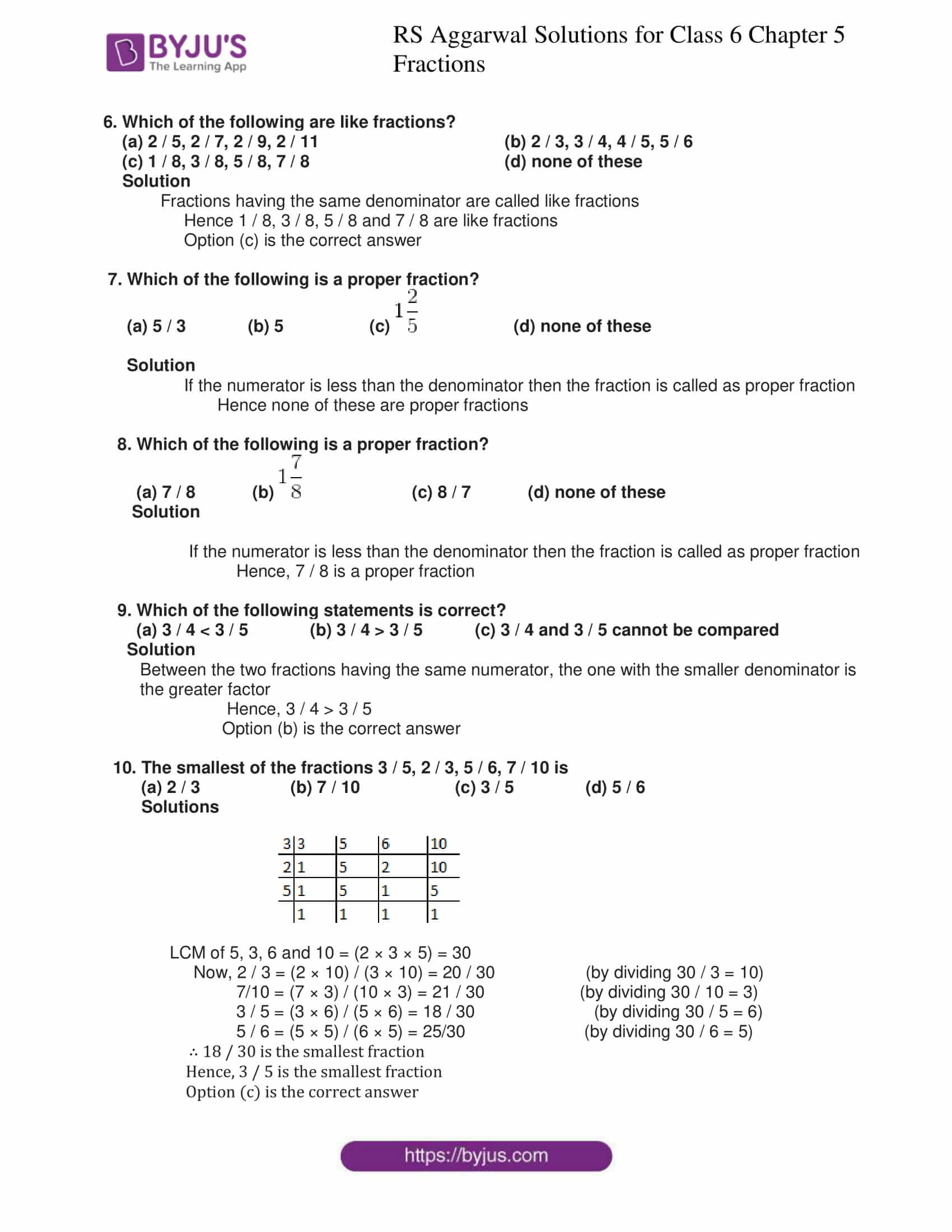### Access answers to Maths RS Aggarwal Solutions for Class 6 Chapter 5 Fractions Exercise 5G

1. A fraction equivalent to 3 / 5 is

(a) 3 + 2 / 5 + 2 (b) 3 – 2 / 5 – 2 (c) 3 × 2 / 5 × 2 (d) none of these

Solution

Since two or more fractions representing the same part of a whole are called equivalent fraction

Thus 3 × 2 / 5 × 2 is equivalent fraction to 3 / 5

2. A fraction equivalent to 8 / 12 is

(a) 8 + 4 / 12 + 4 (b) 8 – 4 / 12 – 4 (c) 8 ÷ 4 / 12 ÷ 4 (d) none of these

Solution

Since two or more fractions representing the same part of a whole are called equivalent fraction

Thus 8 ÷ 4 / 12 ÷ 4 is equivalent fraction to 8 / 12

3. A fraction equivalent to 24 / 36 is

(a) 3 / 4 (b) 2 / 3 (c) 8 / 9 (d) none of these

Solution

1, 2, 3, 4, 6, 8, 12, 24 are the factors of 24

1, 2, 3, 4, 6, 9, 12, 18, 36 are the factors of 36

Common factors of 24 and 36 are 1, 2, 3, 4, 6 and12

HCF = 12

Now dividing both numerator and denominator by 12

= 24 ÷ 12/36 ÷ 12

= 2 / 3

Thus 2 / 3 is the equivalent factor to 24 / 36

4. If 3 / 4 is equivalent to x / 20 then the value of x is

(a) 15 (b) 18 (c) 12 (d) none of these

Solution

Since 3 / 4 = x / 20

20 × 3 = 4x

4x = 60

= 60 / 4

x = 30 / 2

x = 15

Hence, the value of x = 15

Option (a) is the correct answer

5. If 45 / 60 is equivalent to 3 / x then the value of x is

(a) 4 (b) 5 (c) 6 (d) 20

Solution

Since 45 / 60 = 3 / x

45x = 60 × 3

45x = 180

x = 180 / 45

x = 36 / 9

x = 4

Hence, the value of x = 4

Option (a) is the correct answer

6. Which of the following are like fractions?

(a) 2 / 5, 2 / 7, 2 / 9, 2 / 11 (b) 2 / 3, 3 / 4, 4 / 5, 5 / 6

(c) 1 / 8, 3 / 8, 5 / 8, 7 / 8 (d) none of these

Solution

Fractions having the same denominator are called like fractions

Hence 1 / 8, 3 / 8, 5 / 8 and 7 / 8 are like fractions

Option (c) is the correct answer

7. Which of the following is a proper fraction?

(a) 5 / 3 (b) 5 (c)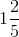(d) none of these

Solution

If the numerator is less than the denominator then the fraction is called as proper fraction

Hence none of these are proper fractions

8. Which of the following is a proper fraction?

(a) 7 / 8 (b)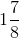(c) 8 / 7 (d) none of these

Solution

If the numerator is less than the denominator then the fraction is called as proper fraction

Hence, 7 / 8 is a proper fraction

9. Which of the following statements is correct?

(a) 3 / 4 < 3 / 5 (b) 3 / 4 > 3 / 5 (c) 3 / 4 and 3 / 5 cannot be compared

Solution

Between the two fractions having the same numerator, the one with the smaller denominator is the greater factor

Hence, 3 / 4 > 3 / 5

Option (b) is the correct answer

10. The smallest of the fractions 3 / 5, 2 / 3, 5 / 6, 7 / 10 is

(a) 2 / 3 (b) 7 / 10 (c) 3 / 5 (d) 5 / 6

Solutions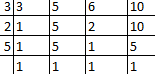LCM of 5, 3, 6 and 10 = (2 × 3 × 5) = 30

Now, 2 / 3 = (2 × 10) / (3 × 10) = 20 / 30 (by dividing 30 / 3 = 10)

7/10 = (7 × 3) / (10 × 3) = 21 / 30 (by dividing 30 / 10 = 3)

3 / 5 = (3 × 6) / (5 × 6) = 18 / 30 (by dividing 30 / 5 = 6)

5 / 6 = (5 × 5) / (6 × 5) = 25/30 (by dividing 30 / 6 = 5)

∴ 18 / 30 is the smallest fraction

Hence, 3 / 5 is the smallest fraction

Option (c) is the correct answer